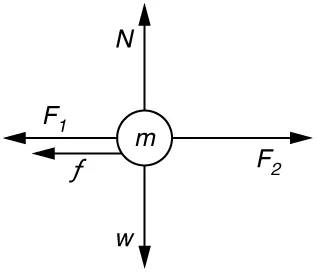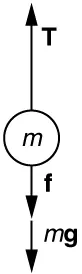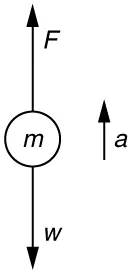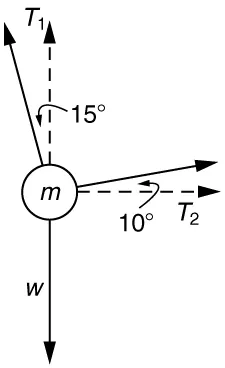College Physics for AP® Courses 2e

# Chapter 4

### Problems & Exercises

1.

265 N

3.

$13.3 m/s 2 13.3 m/s 2$

7.

(a) $12 m/s 2 12 m/s 2$.

(b) The acceleration is not one-fourth of what it was with all rockets burning because the frictional force is still as large as it was with all rockets burning.

9.

(a) The system is the child in the wagon plus the wagon.

(b)(c) $a=0.130 m/s2a=0.130 m/s2$ in the direction of the second child’s push.

(d) $a=0.00 m/s2a=0.00 m/s2$

11.

(a) $3.68 × 10 3 N 3.68× 10 3 N$ . This force is 5.00 times greater than his weight.

(b) $3750 N; 11.3º above horizontal3750 N; 11.3º above horizontal$

13.

$1.5 × 10 3 N , 150 kg , 150 kg 1.5× 10 3 N,150 kg,150 kg$

15.

Force on shell: $2.64×107N2.64×107N$

Force exerted on ship = $−2.64×107N−2.64×107N$, by Newton’s third law

17.

(a) $0.11 m/s2 0.11 m/s2$
(b) $1.2× 10 4 N1.2× 10 4 N$

19.

(a) $7.84×10-4 N7.84×10-4 N$

(b) $1.89×10–3 N1.89×10–3 N$. This is 2.41 times the tension in the vertical strand.

21.

Newton’s second law applied in vertical direction gives

$F y = F − 2 T sin θ = 0 F y = F − 2 T sin θ = 0$
$F = 2T sin θ F = 2T sin θ$
$T = F 2 sin θ . T = F 2 sin θ .$
23.Using the free-body diagram:

$Fnet=T−f−mg=maFnet=T−f−mg=ma$,

so that

$a=T−f−mgm=1.250×107 N−4.50×106 N−(5.00×105 kg)(9.80 m/s2)5.00×105 kg=6.20 m/s2a=T−f−mgm=1.250×107 N−4.50×106 N−(5.00×105 kg)(9.80 m/s2)5.00×105 kg=6.20 m/s2$.

25.
1. Use Newton’s laws of motion.2. Given : $a=4.00g=(4.00)(9.80 m/s2)=39.2 m/s2 ; a=4.00g=(4.00)(9.80 m/s2)=39.2 m/s2 ;$$m=70.0 kgm=70.0 kg$,

Find: $FF$.

3. $∑F=+F−w=ma ,∑F=+F−w=ma ,$ so that $F=ma+w=ma+mg=m(a+g)F=ma+w=ma+mg=m(a+g)$.

$F=(70.0 kg)[(39.2 m/s2)+(9.80 m/s2)]F=(70.0 kg)[(39.2 m/s2)+(9.80 m/s2)]$$=3.43×103 N=3.43×103 N$. The force exerted by the high-jumper is actually down on the ground, but $FF$ is up from the ground and makes him jump.

4. This result is reasonable, since it is quite possible for a person to exert a force of the magnitude of $103 N103 N$.
27.

(a) $4.41×105 N4.41×105 N$

(b) $1.50×105 N1.50×105 N$

29.

(a) $910 N910 N$

(b) $1.11×103 N1.11×103 N$

31.

$a = 0.139 m/sa=0.139 m/s$, $θ = 12.4º θ=12.4º$ north of east

33.
1. Use Newton’s laws since we are looking for forces.
2. Draw a free-body diagram:3. The tension is given as $T=25.0 N.T=25.0 N.$ Find $Fapp.Fapp.$ Using Newton’s laws gives: $Σ Fy=0,Σ Fy=0,$ so that applied force is due to the y-components of the two tensions: $Fapp=2 T sin θ=2(25.0 N)sin(15º)=12.9 NFapp=2 T sin θ=2(25.0 N)sin(15º)=12.9 N$

The x-components of the tension cancel. $∑Fx=0∑Fx=0$.

4. This seems reasonable, since the applied tensions should be greater than the force applied to the tooth.
40.

$10.2 m/s 2 , 4.67º from vertical 10.2 m/s 2 , 4.67º from vertical$

42.$T 1 = 736 N T 1 = 736 N$

$T 2 = 194 N T 2 = 194 N$

44.

(a) $7.43 m/s7.43 m/s$

(b) 2.97 m

46.

(a) $4.20 m/s4.20 m/s$

(b) $29.4 m/s229.4 m/s2$

(c) $4.31×103 N4.31×103 N$

48.

(a) 47.1 m/s

(b) $2.47×103 m/s22.47×103 m/s2$

(c) $6.18×103 N6.18×103 N$ . The average force is 252 times the shell’s weight.

52.

(a) $1×10−131×10−13$

(b) $1×10−111×10−11$

54.

$10 2 10 2$

55.

(a) Box A travels faster at the finishing distance since a greater force with equal mass results in a greater acceleration. Also, a greater acceleration over the same distance results in a greater final speed.

(b) i. Yes, it is consistent because a greater force results in a greater final speed. ii. It does not make sense because $V= 2(f/m)x =K (F) V= 2(f/m)x =K (F)$.

(c)

Figure 4.41

### Test Prep for AP® Courses

1.
Figure 4.44

Car X is shown on the left, and Car Y is shown on the right.

i.

Car X takes longer to accelerate and does not spend any time traveling at top speed. Car Y accelerates over a shorter time and spends time going at top speed. So Car Y must cover the straightaways in a shorter time. Curves take the same time, so Car Y must overall take a shorter time.

ii.

The only difference in the calculations for the time of one segment of linear acceleration is the difference in distances. That shows that Car X takes longer to accelerate. The equation $d4vc=tcd4vc=tc$ corresponds to Car Y traveling for a time at top speed.

Substituting $a=vct1a=vct1$ into the displacement equation in part (b) ii gives $D=32vct1D=32vct1$. This shows that a car takes less time to reach its maximum speed when it accelerates over a shorter distance. Therefore, Car Y reaches its maximum speed more quickly, and spends more time at its maximum speed than Car X does, as argued in part (b) i.

3.

A body cannot exert a force on itself. The hawk may accelerate as a result of several forces. The hawk may accelerate toward Earth as a result of the force due to gravity. The hawk may accelerate as a result of the additional force exerted on it by wind. The hawk may accelerate as a result of orienting its body to create less air resistance, thus increasing the net force forward.

5.

(a) A soccer player, gravity, air, and friction commonly exert forces on a soccer ball being kicked.

(b) Gravity and the surrounding water commonly exert forces on a dolphin jumping. (The dolphin moves its muscles to exert a force on the water. The water exerts an equal force on the dolphin, resulting in the dolphin’s motion.)

(c) Gravity and air exert forces on a parachutist drifting to Earth.

7.

(c)

9.
Figure 4.45

The diagram consists of a black dot in the center and two small red arrows pointing up (Fb) and down (Fg) and two long red arrows pointing right (Fc = 9.0 N) and left (Fw=13.0 N).

In the diagram, Fg represents the force due to gravity on the balloon, and Fb represents the buoyant force. These two forces are equal in magnitude and opposite in direction. Fc represents the force of the current. Fw represents the force of the wind. The net force on the balloon will be $Fw−Fc=4.0Fw−Fc=4.0$ N and the balloon will accelerate in the direction the wind is blowing.

11.

Since $m=F/am=F/a$, the parachutist has a mass of .

For the first 2 s, the parachutist accelerates at 9.8 m/s2.

$v = a t = 9.8 m s 2 • 2 s = 19.6 m s v = a t = 9.8 m s 2 • 2 s = 19.6 m s$

Her speed after 2 s is 19.6 m/s.

From 2 s to 10 s, the net force on the parachutist is 539 N – 615 N, or 76 N upward.

$a = F m = − 76 N 55 ​ ​ ​ ​ kg = − 1.4 m s 2 a = F m = − 76 N 55 ​ ​ ​ ​ kg = − 1.4 m s 2$

Since $v=v0+atv=v0+at$, $v=19.6 m/s2+(−1.4 m/s2)(8s) =8.4 m/s2v=19.6 m/s2+(−1.4 m/s2)(8s) =8.4 m/s2$.

At 10 s, the parachutist is falling to Earth at 8.4 m/s.

13.

The system includes the gardener and the wheelbarrow with its contents. The following forces are important to include: the weight of the wheelbarrow, the weight of the gardener, the normal force for the wheelbarrow and the gardener, the force of the gardener pushing against the ground and the equal force of the ground pushing back against the gardener, and any friction in the wheelbarrow’s wheels.

15.

The system undergoing acceleration is the two figure skaters together.

Net force = $120 N – 5.0 N = 115 N120 N – 5.0 N = 115 N$.

Total mass = .

Using Newton’s second law, we have that

$a = F m = 115 N 90 kg = 1.28 m s 2 a = F m = 115 N 90 kg = 1.28 m s 2$

The pair accelerates forward at 1.28 m/s2.

17.

The force of tension must equal the force of gravity plus the force necessary to accelerate the mass. $F=mgF=mg$ can be used to calculate the first, and $F=maF=ma$ can be used to calculate the second.

For gravity:

$F = m g = ( 120.0 kg)(9 .8 m/s 2 ) = 1205.4 N F = m g = ( 120.0 kg)(9 .8 m/s 2 ) = 1205.4 N$

For acceleration:

$F = m a = ( 120.0 kg)(1 .3 m/s 2 ) = 159.9 N F = m a = ( 120.0 kg)(1 .3 m/s 2 ) = 159.9 N$

The total force of tension in the cable is 1176 N + 156 N = 1332 N.

19.

(b)

21.
Figure 4.47

The diagram has a black dot and three solid red arrows pointing away from the dot. Arrow Ft is long and pointing to the left and slightly down. Arrow Fw is also long and is a bit below a diagonal line halfway between pointing up and pointing to the right. A short arrow Fg is pointing down.

Fg is the force on the kite due to gravity.

Fw is the force exerted on the kite by the wind.

Ft is the force of tension in the string holding the kite. It must balance the vector sum of the other two forces for the kite to float stationary in the air.

23.

(b)

25.

(d)

27.

A free-body diagram would show a northward force of 64 N and a westward force of 38 N. The net force is equal to the sum of the two applied forces. It can be found using the Pythagorean theorem:

$F net = F x 2 + F y w = ( 38 N ) 2 + ( 64 ​ N ) 2 = 74.4 N F net = F x 2 + F y w = ( 38 N ) 2 + ( 64 ​ N ) 2 = 74.4 N$

Since $a=Fma=Fm$,

$a = 74.4 N 825 kg = 0.09 m/s 2 a = 74.4 N 825 kg = 0.09 m/s 2$

The boulder will accelerate at 0.09 m/s2.

29.

(b)

31.

(b)

33.

(d)

Order a print copy

As an Amazon Associate we earn from qualifying purchases.